# Inverse mapping

(diff) ← Older revision | Latest revision (diff) | Newer revision → (diff)

(inverse operator) of a single-valued onto mapping (operator)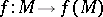A single-valued mappingsuch that(1)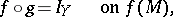(2)

where,andare any sets.

Ifsatisfies only condition (1), then it is called a left-inverse mapping of, and if it satisfies only condition (2), it is a right-inverse mapping of. The inverse mapping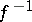exists if and only if for eachthe complete inverse image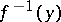consists of a single element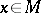. Ifhas an inverse mapping, then the equation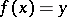(3)

has a unique solution for each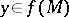. If only a right inverse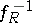exists, then a solution of (3) exists, but its uniqueness is an open question. If only a left inverseexists, then the solution is unique, assuming that it exists. Ifandare vector spaces and ifis a linear operator frominto, thenis also linear, if it exists. In general, ifandare endowed with some kind of structure, it may happen that certain properties ofare also inherited by, assuming that it exists. E.g., ifandare Banach spaces andis a closed operator, then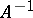is also closed; ifis a Hilbert space andis self-adjoint, thenis also self-adjoint; ifis an odd function, thenis also odd, etc. The continuity ofdoes not always imply the continuity offor many important classes of linear operators, for example for completely-continuous operators. The following are important tests for the continuity of the inverse of a linear operator.

Letbe a finite-dimensional vector space, with a certain basis, and let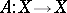be given by the matrixwith respect to this basis. Thenexists if and only if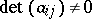(in this caseandare automatically continuous).

Letandbe Banach spaces, and letbe a continuous linear operator frominto.

1) If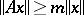, where, thenexists and is continuous.

2) If,, thenexists, is continuous andwhere the series on the right-hand side converges in the norm of the space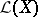.

3) The operatorexists and is continuous on all ofif and only if the conjugate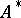has an inverse which is defined and continuous on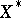. Here.

4) Ifexists, is continuous and if, then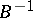also exists, is continuous and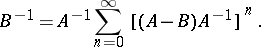Thus, the set of invertible operators is open inin the uniform topology of this space.

5) Banach's open mapping theorem: Ifis a one-to-one mapping ofonto, then the inverse mapping, which exists, is continuous. This theorem has the following generalization: A one-to-one continuous linear mapping of a fully-complete spaceonto a separated barrelled spaceis a topological isomorphism.

The spectral theory of linear operators on a Hilbert space contains a number of results on the existence and continuity of the inverse of a continuous linear operator. E.g., ifis self-adjoint andis not real, then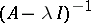exists and is continuous.

How to Cite This Entry:
Inverse mapping. Encyclopedia of Mathematics. URL: http://encyclopediaofmath.org/index.php?title=Inverse_mapping&oldid=15880
This article was adapted from an original article by V.I. Sobolev (originator), which appeared in Encyclopedia of Mathematics - ISBN 1402006098. See original article